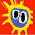## Wednesday, December 16, 2009

### By-name-parameter to Function

Today's topic is related to Defining Custom Control Structures. By name parameters are not function objects in the same way as other functions. Normally you need to invoke a function: `scala> def exec(func: () => Int) = func()exec: (() => Int)Int// exec invokes the function so 10 is the resultscala> exec (() => 10)  res1: Int = 10scala> def exec(func: () => Int) = funcexec: (() => Int)() => Int// here exec returns the function:scala> exec (() => 10)                 res2: () => Int = <function>`
Compare that to a by-name-parameter function:
`scala> def exec(func: => Int) = func   exec: (=> Int)Int// by-name-parameters are executed when they are referenced// so the result is 10scala> exec {10}                    res3: Int = 10// This is not legal because by-name-parameters// are not normal functionsscala> def exec(func: => Int) = func()<console>:4: error: func of type Int does not take parameters       def exec(func: => Int) = func()`
So the issue is how can you pass a by-name-parameter to a method that takes a function as a parameter without having to do:
`scala> def takesFunc(func: () => Int) = println(func())takesFunc: (() => Int)Unitscala> def send(x: => Int) = takesFunc(() => x) send: (=> Int)Unitscala> send{2}2`
the alternative syntax is:
`scala> def send(x: => Int) = takesFunc (x _)   send: (=> Int)Unitscala> send {2}2`

1.2.3.